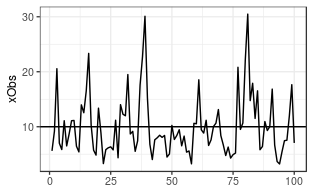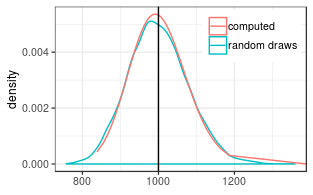# Aggregating a series of correlated lognormal variables

## Generating observations and log-normally distributed random errors

We generate 10000 Observations of a sum of 100 random variables with mean 10 and multiplicative standard deviation of 1.7.

nObs <- 100; nRep <- 10000
#nObs <- 1000; nRep <- 100
xTrue <- rep(10, nObs)
sigmaStar <- rep(1.7, nObs) # multiplicative stddev
theta <- getParmsLognormForExpval(xTrue, sigmaStar)
# generate observations with correlated errors
acf1 <- c(0.4,0.1)
corrM <- setMatrixOffDiagonals(
diag(nrow = nObs), value = acf1, isSymmetric = TRUE)
xObsN <- exp(mvtnorm::rmvnorm(
nRep, mean = theta[,1]
, sigma = diag(theta[,2]) %*% corrM %*% diag(theta[,2])))

A single draw of the autocorrelated 100 variables looks like the following.

##    Min. 1st Qu.  Median    Mean 3rd Qu.    Max.
##   757.5   945.0   996.1   999.3  1050.2  1359.1## Estimating the correlation matrix and effective number of parameters

The original autocorrelation function used to generate the sample was:

c(1, acf1)
##  1.0 0.4 0.1

The effective autocorrelation function estimated from the sample is:

(effAcf <- computeEffectiveAutoCorr(ds$xErr)) ##  1.00000000 0.42254489 0.13839939 0.01567392 (nEff <- computeEffectiveNumObs(ds$xErr))
##  46.76592

Due to autocorrelation, the effective number of parameters is less than nObs = 100.

## Computing the mean and its standard deviation

First we compute the distribution parameter of the sum of the 100 variables. The multiplicative uncertainty has decreased from 1.7.

#coefSum <- estimateSumLognormal( theta[,1], theta[,2], effAcf = effAcf )
coefSum <- estimateSumLognormal( theta[,1], theta[,2], effAcf = c(1,acf1) )
exp(coefSum["sigma"])
##    sigma
## 1.077687

Its expected value corresponds to the expected value (100*10).

(sumExp <- getLognormMoments( coefSum, coefSum)[1,"mean"])
## mean
## 1000

The lognormal approximation of the distribution of the sum, is close to the distribution of the 10000 repetitions.